## She Loves Data: R Workshop Day 1 – ListLists are straightforward type of data structure, by using list().

Syntax: list(vector, val2, …)
Lists:
– Store an ordered collections of objects.
– It can contain different data types, works like a container without restriction.

`#Define a list with vector, floating number and function, sin.list <- list(c(2,3,5), 31.7, sin)`

The output on my console shows,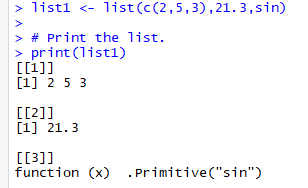Three different elements list on the console.
Another example of using list was in my previous blog on Matrix, where I define the dimnames using list in the example.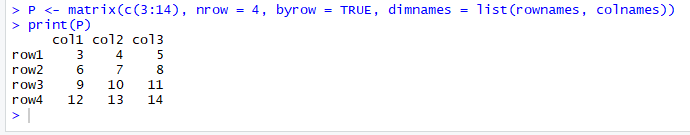The rownames and colnames are vectors which contain the names of the rows and columns.

I do not have many example using list() especially on how to access the elements of the list. However, I believe it should be able to retrieve the elements similarly to other programming languages by using the indexes and square brackets. I will give an update on that later.

## She Loves Data: R Workshop Day 1 – Data FrameIt is an important data structure in R.

Syntax: data.frame() everything will be declared within the parenthesis.
Data Frames:
– Generated by combining multiple vectors
– It can be created by using external files when importing the data into R.

I am not sure how to share what I learned about data frame in just one blog entry. It works slightly different than matrices, where data frame can contain different modes of data. See example below:

`#Create the data frame. emp.data <- data.frame(   emp_id = c(1:5),    emp_name = c("Rick","Dan","Michelle","Ryan","Gary"),   salary = c(623.3,515.2,611.0,729.0,843.25),  start_date = as.Date(c("2012-01-01", "2013-09-23", "2014-11-15", "2014-05-11","2015-03-27")) )#Print the data frame. print(emp.data) `

A data frame is created called emp.data which contains of number for emp_id, characters for emp_name, floating points for salary and date for start working date. The output of the data frame on the console when I print(emp.data) is as below: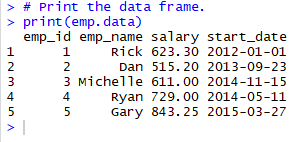In data frame, the column names are taken from the variable names of the vectors.

Data frame has several built-in R functions which are quite useful. Follow the examples below:

str(emp.data)
– When I execute the above code, the console shows:
‘data.frame’: 5 obs. of 4 variables:
\$ emp_id : int 1 2 3 4 5
\$ emp_name : Factor w/ 5 levels “Dan”,”Gary”,”Michelle”,..: 4 1 3 5 2
\$ salary : num 623 515 611 729 843
\$ start_date: Date, format: “2012-01-01” “2013-09-23” “2014-11-15” “2014-05-11” …

Do you know why it is 5 objects? Yes, 4 vectors and a data frame.

View(emp.data)
– View the data in tabular format.
– Navigate to the top left box in the RStudio, I see another tab named with empdata displayed.
– Use it often to check or view data.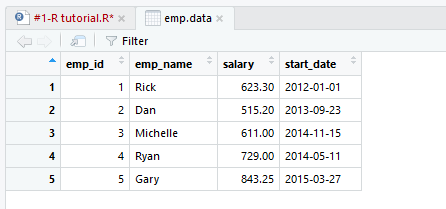Cool, right?

Next cool things we can do with data frame is using the summary(emp.data).
– Print out the summary and it shows the min, max, median, mean, 1st quarter and 3rd Quarter. In some statistics analysis, this is very useful piece of information.
– How to do extract just min, median and max values from the summary()?

What if I want to extract specific columns from the data frame? How does it can be done? Below codes explain and the output on the console. I can access to the columns in the data frame by using “\$” symbol.

`#Extract Specific columns. result <- data.frame(emp.data\$emp_name,emp.data\$salary) print(result)`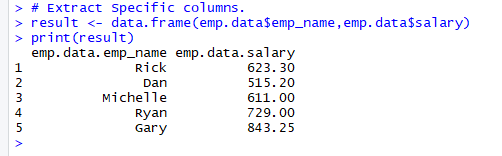Accessing the data frame.
– Extract information of a specific rows and columns.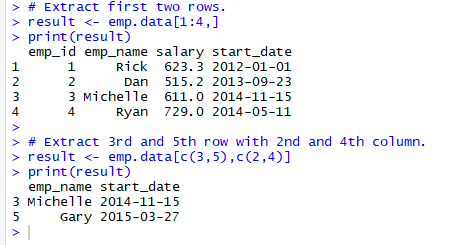– Extract using head() and tail().
In a larger data frame, it is quite useful function to extract top 6 records and last 6 records. The example from the workshop is not large enough to see the different, so let try head(mtcars) and tail(mtcars).

mtcars is built-in data frame in RStudio.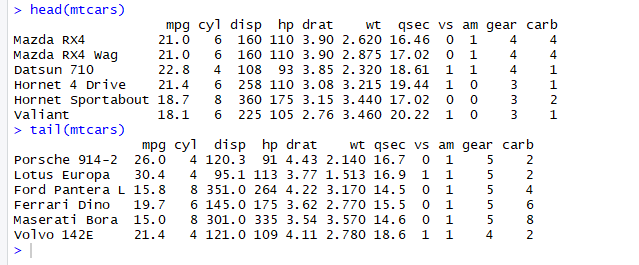To add another “column”, it can be done directly with codes below:
emp.data\$dept < – c(“IT”,”Operations”,”IT”,”HR”,”Finance”).

Then, map it to a variable to print out on the console using the following codes,

`#Add the "dept" column. emp.data\$dept <- c("IT","Operations","IT","HR","Finance") v <- emp.data print(v)`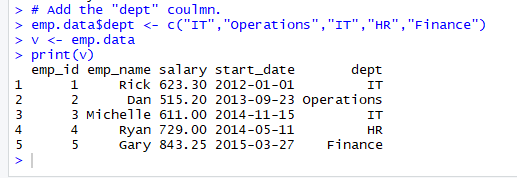The key is using “\$“, the same key I used to extract or access data from emp.data data frame.

I will share more on data frames when I come across interesting codes.  Stay tuned. Thank you.

## She Loves Data: R Workshop Day 1 – MatrixI continue with the next data structure in R language, let have a quick zoom into matrices with example given by the instructor as below using the matrix syntax:

Syntax : matrix(data, nrow, ncol, byrow, dimnames)

Matrices:
– Elements are arranged sequentially by row.
– It starts with row, then column.
data can be a form of vector.
nrow or ncol means desired number of rows or columns.
byrow is a logical value, TRUE or FALSE. By default, the matrix is filled by columns, otherwise the matrix is filled by rows.

Let me share the examples from the workshop.

`#matrices #Elements are arranged sequentially by row. M <- matrix(c(3:15), nrow = 4, byrow = TRUE) print(M) #Elements are arranged sequentially by column. N <- matrix(c(3:14), nrow = 4, byrow = FALSE) print(N)`

My output on the console,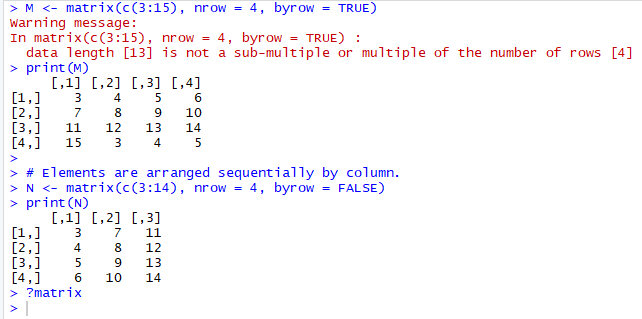Notice the above warning message. It is because the length of the vector c ranging from number 3 to 15, inclusive, is only 13 and I wanted to create a 4 rows matrix (m) by setting nrow = 4, byrow = TRUE.

However, if I changed byrow = FALSE, the 13 elements are arranged by column and returned the matrix (n) without warning message.

Rename rows and columns in matrix
It allows to define the row and column names using dimnames.

`#Define the column and row names. rownames = c("row1", "row2", "row3", "row4") colnames = c("col1", "col2", "col3") P <- matrix(c(3:14), nrow = 4, byrow = TRUE, dimnames = list(rownames, colnames)) print(P)`Rename the row and column names gives us a better picture of the matrix.

Before I move on, do you notice the above codes contains vector, matrix and list? Amazing, right?

Does Python or Scala able to do so? Please share with me if you know it.

Access elements in matrix
I can access the element in the matrix using index, which begin with 1. Some example using the matrix (p) to find some values.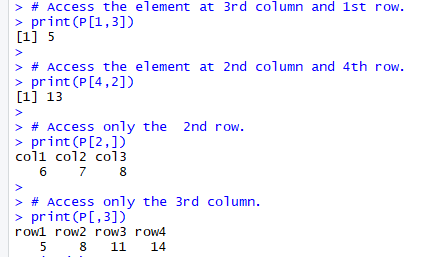Matrix of the same data type and number of elements within a matrix, can perform addition of both matrix together or subtraction values between the matrices.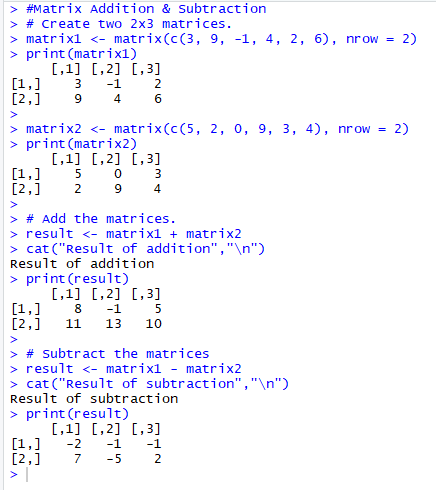Pretty cool right, working around with matrices. I am going to share two more data structures, the list and the data frame in my next blogs.

## She Loves Data: R Workshop Day 1 – VectorsAfter a short break over the weekend, I began my learning again. Let continue exploring the data structure in R language, the vectors. The basic ones to play around and get familiar with codes.

Syntax: c(val1, val3, val3, …)

Vectors
– Simplest, basic data structure in R.
– Contains same type of data.

`#Vector declaration#vector containing three numeric values 2, 3 and 5. c(2, 3, 5)#vector contains 1 to 10. First value before colon (:) is start number, and value after the colon is end number.c(1:10)#vector of logical values.  c(TRUE, FALSE, TRUE, FALSE, FALSE)  #vector of character values.c("aa", "bb", "cc", "dd", "ee") #Check the length of the vector and it returns 5.length(c("aa", "bb", "cc", "dd", "ee")) #check the length of a value, "aa" in the vector. It returns 2.nchar( c("aa")) #check the data type,n = c("aa", "bb", "cc", "dd", "ee")  class(n) "character"typeof(n) "character" #Full codes:   c("aa", "bb", "cc", "dd", "ee")     "aa" "bb" "cc" "dd" "ee"   length(c("aa", "bb", "cc", "dd", "ee"))    5   nchar( c("aa"))    2   n = c("aa", "bb", "cc", "dd", "ee")    class(n)    "character"   typeof(n)    "character"  #Combining vectors. It converts the numeric into string.   n = c(2, 3, 5)    s = c("aa", "bb", "cc", "dd", "ee")    c(n, s)    "2"  "3"  "5"  "aa" "bb" "cc" "dd" "ee"   `

I learned from the recent R workshop organized by Sparkline, the vectors can be used in many different situations. I will try to point it out whenever I share the codes in my next blogs. Let us move on to the next data structure.

## She Loves Data: R Workshop Day 1 – Basic Data Type ConversionIt works similarly to other programming languages, in R, it has.
– use is.xxx() to check the data type is of ‘xxx’ type and returns TRUE or FALSE.
– use as.xxx() to convert it into ‘xxx’ type.

Example,

• is.numeric(), is.integer(), is.character(), is.vector(), is.matrix(), is.data.frame()
• as.numeric(), as.integer(), as.character(), as.vector(), as.matrix(), as.data.frame)

I have tried to use as.integer() previously, still remember?

` #When I execute the line, y = as.integer(3), the output is,   y = as.integer(3)   class(y)    "integer"  `

With as.integer, it converts the “3” into integer data type and when use the class(y) it returns integer. Another one which can be used quite often is as.character(), convert a number into a string.

More data conversion is showed in the chart below, it is taken from, https://www.statmethods.net/management/typeconversion.html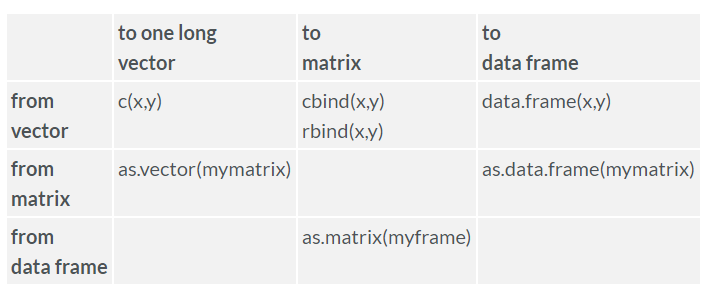After talking so much for character, numeric, string and Boolean, how about Date? Do we able to convert date?

Answer is yes. It has as.date() to convert character into date with default format, yyyy-mm-dd. Of course, we can change the date format. Example, it could be coded as,

as.Date(x, “format”)

where the date formatting can refer to below table,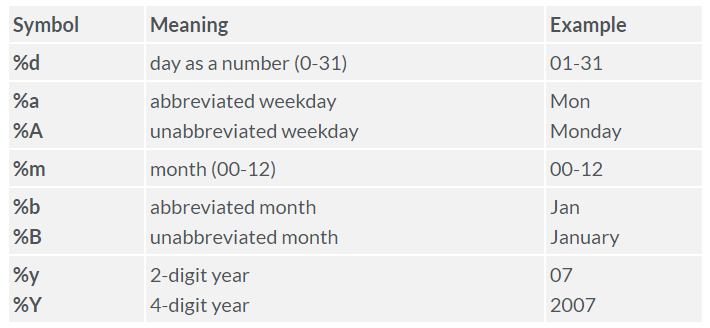Right now, I do not have much examples to show data conversion and formatting. Hopefully in the future posts, there will be more sample codes written with data conversion or formatting.

## She Loves Data: R Workshop Day 1 – Basic Data StructureComes into the most important part of R, the data structure or data objects in R. It holds data and it is used to handle all computations in R. Let explore one by one. It is a bit theory for this portion. It gives some information of what is vectors, matrices, lists and data frames.

Vector : c(val1, val3, val3, …)
– Simplest, basic data structure in R.
– Contains same type of data.

Matrix : matrix(data, nrow, ncol, byrow, dimnames)
– Can do operations such as addition, multiplication on matrix.
– Elements are arranged sequentially by row.
– It starts with row, then column.
data can be a form of vector.
nrow or ncol means desired number of rows or columns.
byrow is a logical value, TRUE or FALSE. By default, the matrix is filled by columns, otherwise the matrix is filled by rows.

List : list(vector, val2, …)
– Store an ordered collections of objects. It allows me to gather a variety of possibly unrelated objects under one name.
– It can contain different data types, works like a container without restriction.
– Declares using list() function or coerce an object using as.list().

Data Frames : data.frame()
– Generated by combining multiple vectors, such that each vector becomes a separate column.
– A very important data structure in R.
– It can be created by using external files when importing the data into R, looks similar like a tabular data objects.
– It can be converted into a matrix by using as.matrix().

I have prepared separate blogs to go into each of them with sample codes I got from the workshop and online learning website.

## She Loves Data: R Workshop Day 1 – Basic Data TypeContinuing from the previous blog, I am going to share some examples for the six data type. These examples can be found from the online learning website, https://www.tutorialspoint.com/r/r_data_types.htm. To help myself to confirm what kind of object is it and what is the object’s data type, the use of class() and type() help to print out the class name of the variable.

Character
– Using single quote or double quote will be fine.
– ‘a’, “good”, “EXAMPLE”, “TRUE”, “3.14”

` #When I execute the line, x = "TRUE", the output is,   x = "TRUE"   class(x)    "character" `

Numeric
– It can be whole number or with decimal points.
– 12.3, 10, 999.
– At the global environment tab, I see the value of x is 10.

`#When I execute the line, x = 10, the output is,   x = 10   class(x)    "numeric" `

Integer
– To declare an integer, I need to use as.integer().
– It converts the value into Integer data type, only keeping the whole number.
– At the global environment tab, I see a different representation of Integer compare to Numeric.

`#When I execute the line, y = as.integer(3), the output is,   y = as.integer(3)   class(y)    "integer" #When I execute the line, z = as.integer(3.14), the output is,   z = as.integer(3.14)   class(z)    "integer"    z    3 `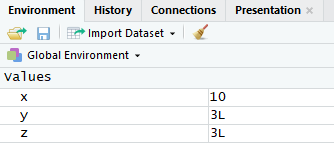There is a “L” behind the number to represent it is a Integer.
Hope it is not too confused for Numeric and Integer. If you have some database SQL background, these can easily be understood as well.

Logical
– It has only two returns values either, TRUE, FALSE.
– It can compare with two variables.
– The standard logical operations are “&” (AND), “|” (OR) and “!” (Negation).

`#When I execute a code that reads,   x = 1; y = 2   # sample values    z = x > y      # is x larger than y?    z              # print the logical value     FALSE   class(z)    "logical"  #This is one of the example of comparison of two values and check if one of them larger than the other one. #If I want to check with 3 variables with declaring z = 5, then assign the logical value to a, then it goes as, a = x > y > z. However, I received an error message,   x = 1; y = 2; z = 5   # sample values    a = x > y > z   Error: unexpected '>' in "a = x > y >" #Can I know why? #Another example to demonstrate the logical operations.   u = TRUE; v = FALSE    u & v    FALSE   u | v       TRUE   !u    FALSE `

Complex
– It refers to complex numbers with real and imaginary parts.
– I do not have example to execute on this part. Will update it next time when I encounter one good example to share.

Raw
– I did not see it elsewhere for an example or what it is about. Anyone can share?

I was a little confused when most people refer R Data Type as vector, matrix, lists and data frame. Yes, it is data type in R, and in general view, it may known as data structure, a collection of objects whereas object’s data type can be character, numeric, integer and Boolean.

R provides us many functions to check the objects, such as

• class() = what kind of object is it?
• typeof() = what is the object’s data type?
• length() = how long is it?
• attributes() = does it have any metadata?

So far, I am able to use class() and hopefully will have a chance to try the rest of the above.

Then how about date? Is it also a data type?

Dates are represented as the number of days since 1970-01-01, with negative values for earlier dates.

Two built-in R functions for dates,

• Sys.Date() returns today’s date.
• date() returns the current date and time.